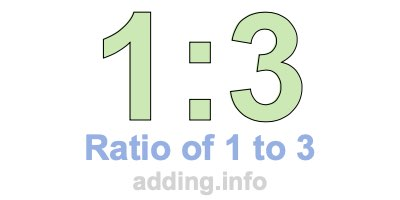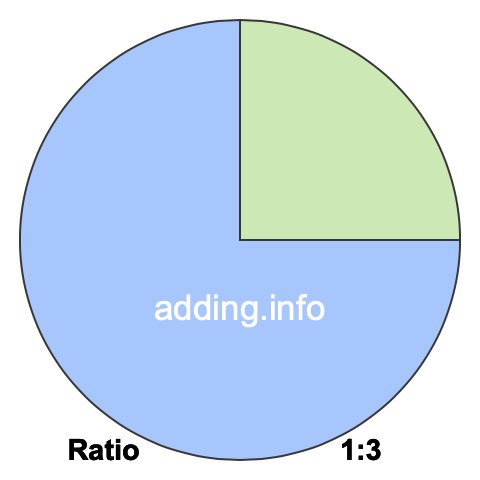Ratio of 1 to 3A ratio of 1 to 3 can be written as 1 to 3, 1:3, or 1/3. Furthermore, 1 and 3 can be the quantity or measurement of anything, such as students, fruit, weights, heights, speed and so on.

A ratio of 1 to 3 simply means that for every 1 of something, there are 3 of something else, with a total of 4.

In the box below, you can enter a number in either box and we will calculate the other number to keep the ratio of 1 to 3. In other words, we will calculate the corresponding equivalent ratio of 1:3 for you.

:

To calculate the left side of the ratio, our calculator multiplies the right side by 1 and then divides the product by 3. To calculate the right side of the ratio, our calculator multiplies the left side by 3 and then divides the product by 1. Answers are rounded to two decimals if necessary.

Below is a list of equivalent ratios of 1:3 that we created using our 1:3 ratio calculator above.

1:3

2:6

3:9

4:12

5:15

6:18

7:21

8:24

9:27

10:30

11:33

12:36

We have also created a pie chart for you to put 1:3 ratio in perspective. To do so, we divided a pie into 4 pieces and then colored 1 pieces green and 3 pieces blue. The pie will look exactly the same using any equivalent ratio of 1:3.The green part of the pie covers 25% and the blue part covers 75%. To calculate the percentages, we divided each side of the ratio by its total and then multiplied by 100, like this:

(1 / 4)×100 = 25
(3 / 4)×100 = 75

In fractional terms, 1/3 of the pie above is colored in green. This means that the ratio of 1 to 3 or 1:3 can also be expressed as a fraction, where the 1 to the left of the colon is the numerator and the 3 to the right of the colon is the denominator:

1:3 = 1/3

Ratio is often used when defining rectangles in width:height format. Width and height can be feet, inches, centimeters, or any other kind of length. With a ratio of 1 to 3, the width is 1 and the height is 3. Below is a picture of what a rectangle with a ratio of 1:3 looks like.Since the simplest form of the fraction 1/3 is 1/3, the simplest form of the ratio 1:3 is also 1:3.

Ratio Calculator
Need information about another ratio? Here you can submit another ratio for us to explain.

:

Ratio of 1 to 4
Go to the next ratio on our list that we have detailed information about.